# Engineering Mathematics: Fourier Series Formula pdf

An online fourier series formulas printable

## 1. Fourier Series – Introduction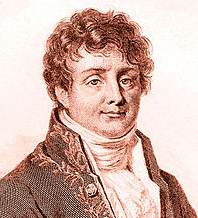Jean Baptiste Joseph Fourier (1768-1830) was a French mathematician, physicist and engineer, and the founder of Fourier analysis.Fourier series are used in the analysis of periodic functions. The Fourier transform and Fourier’s law are also named in his honour.

### 2. Fourier Series of Even and Odd Functions

A function f(x) is said to be even if f(-x) = f(x).

The function f(x) is said to be odd if f(-x) = -f(x)

Graphically, even functions have symmetry about the y-axis,whereas odd functions have symmetry around the origin.Examples:

Sums of odd powers of x are odd: 5x3– 3x

Sums of even powers of x are even: -x6 + 4x4+ x2-3

sin x is odd, and cos x is even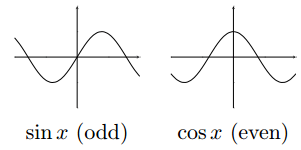The product of two odd functions is even: x sin x is even

The product of two even functions is even: x2cos x is even

The product of an even function and an odd function is odd: sin x cos x is odd

### 3. Integrating even functions over symmetric domains.

Let p > 0 be any fixed number. If f(x) is an odd function, then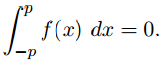Intuition: The area beneath the curve on [-p, 0] is the same as the area under the curve on [0, p], but opposite in sign. So, they cancel each other out!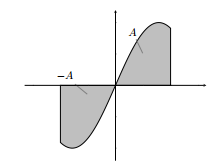Let p > 0 be any fixed number. If f(x) is an even function, then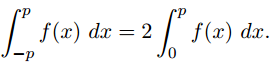Intuition: The area beneath the curve on [-p, 0] is the same as the area under the curve on [0, p], but this time with the same sign. So, you can just find the area under the curve on [0, p] and double it!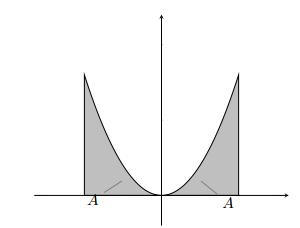### 4. Periodic functions

Definition:

A function f(x) is said to be periodic if there exists a number

T > 0 such that f(x + T) = f(x) for every x. The smallest such

T is called the period of f(x).

Intuition: periodic functions have repetitive behavior.A periodic function can be defined on a finite interval,

then copied and pasted so that it repeats itself.

### 5. The fourier series of the function f(x)a(k) =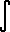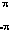f(x) cos kx dx
b(k) =f(x) sin kx dx

### 6. Remainder of fourier series

Sn(x) = sum of first n+1 terms at x.

remainder(n) = f(x) – Sn(x) =f(x+t) Dn(t) dt

Sn(x) =f(x+t) Dn(t) dt

Dn(x) = Dirichlet kernel =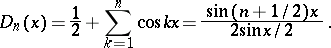The Dirichlet kernel is also called the Dirichlet summation kernel. There is also a different normalization in use: the kernels Dn and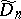are often multiplied by 2. They are then represented also by the series### 7. Riemann’s Theorem

.
If f(x) is continuous except for a finite # of finite jumps in every finite interval then:

lim(k->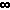)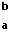f(t) cos kt dt = lim(k->)f(t) sin kt dt = 0

The fourier series of the function f(x) in an arbitrary interval.

A(0) / 2 +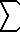(k=1..) [ A(k) cos (k(Π)x / m) + B(k) (sin k(Π)x / m) ]

a(k) = 1/m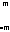f(x) cos (k(Π)x / m) dx

b(k) = 1/mf(x) sin (k(Π)x / m) dx

### 8. Parseval’s Theorem

.

Parseval’s theorem usually refers to the result that the Fourier transform is unitary, that the sum (or integral) of the square of a function is equal to the sum (or integral) of the square of its transform.

If f(x) is continuous; f(-PI) = f(PI) thenf2(x) dx = a(0)2 / 2 +(k=1..) (a(k)2 + b(k)2)

Fourier Integral of the function f(x)

f(x) =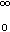( a(y) cos yx + b(y) sin yx ) dy

a(y) =f(t) cos ty dt

b(y) =f(t) sin ty dt

f(x) =dyf(t) cos (y(x-t)) dt

### 9. Special Cases of Fourier Integral

if f(x) = f(-x) then

f(x) =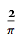cos xy dyf(t) cos yt dt

if f(-x) = -f(x) then

f(x) =sin xy dysin yt dt

### 10. The Fourier Transforms

Fourier Cosine Transform

g(x) =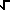(f(t) cos xt dt

Fourier Sine Transform

g(x) =(f(t) sin xt dt

### 11. Identities of the Transforms

If f(-x) = f(x) then

Fourier Cosine Transform ( Fourier Cosine Transform (f(x)) ) = f(x)

If f(-x) = -f(x) then

Fourier Sine Transform (Fourier Sine Transform (f(x)) ) = f(x)